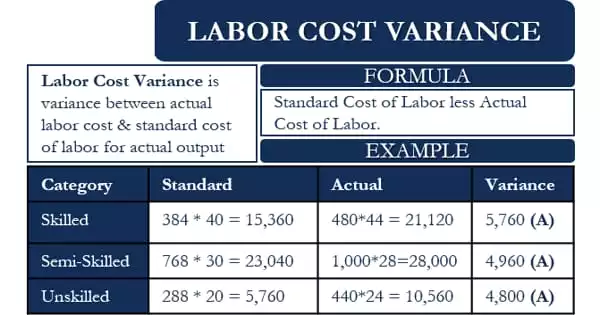Accounting

# Concept of Labor Cost Variance (LCV)The difference between the standard direct labor cost for the actual production and the actual labor cost paid is referred to as direct labor cost variation. It is the discrepancy between the standard labor cost for actual output and the actual labor cost for production. The labor cost variance is defined as the difference between the actual direct wages paid and the direct wages stipulated for the standard output. This variation is also known as wage variance or LCV. The LCV, like other types of variation, can be beneficial or unfavorable.

Formula for calculation of labor cost variance (LCV):

LCV = (ST x SR) – ( AT x AR)

Where,

• LCV = Labor cost variance
• ST = Standard time
• SR = Standard rate
• AT = Actual time
• AR = Actual rate

Labor cost variance represents the total of the labor rate variance and the labor efficiency variance.

Therefore, LCV = Labor rate variance (LRV) + Labor efficiency variance LEV)

If the resulting figure is positive, the difference is denoted by F i.e. favorable variance and if the resulting figure is negative, the difference is denoted by U i.e. unfavorable variance.

Example of Labor Cost Variance

Here we will take up one example for better understanding and clarity about the calculation for Labor Cost Variance.

Company A estimates that it could produce 15,000 units of X using 400 hours of skilled labor, 800 hours of semi-skilled labor, and 300 hours of Unskilled labor. The standard cost of the three types of labor is \$40, \$30, and \$20, respectively.

In reality, Company A was able to produce 14,400 units of X using 480 hours of skilled labor, 1000 hours of semi-skilled labor, and 440 hours of Unskilled labor. The actual cost of the three types of labor is \$44, \$28, and \$24, respectively.

To calculate the LCV we first need to find the standard labor hours for the actual output.

For this, we need to multiply the standard labor hours with the ratio of Actual output and standard output.

This ratio will = 14,400/ 15,000 = 0.96

Now, the standard skilled labor hours for the actual output will be = 400 * 0.96 = 384

The standard semi-skilled labor hours for the actual output will be = 800 * 0.96 = 768

The standard unskilled labor hours for the actual output will be = 300 * 0.96 = 288

Now, we will calculate the Labor Cost Variance for each type of labor.

The LCV for Skilled labor = (384 * \$40) less (480 * \$44) = \$15,360 less \$21,120 = -\$5,760 Adverse

LCV for semi-Skilled labor = (768 * \$30) less (1000 * \$28) = \$23,040 less \$28,000 = – \$4,960 Adverse

LCV for un-Skilled labor = (288 * \$20) less (440 * \$24) = \$5,760 less \$10,560 = – \$4800 Adverse

So, total LCV = –15,520 Adverse.